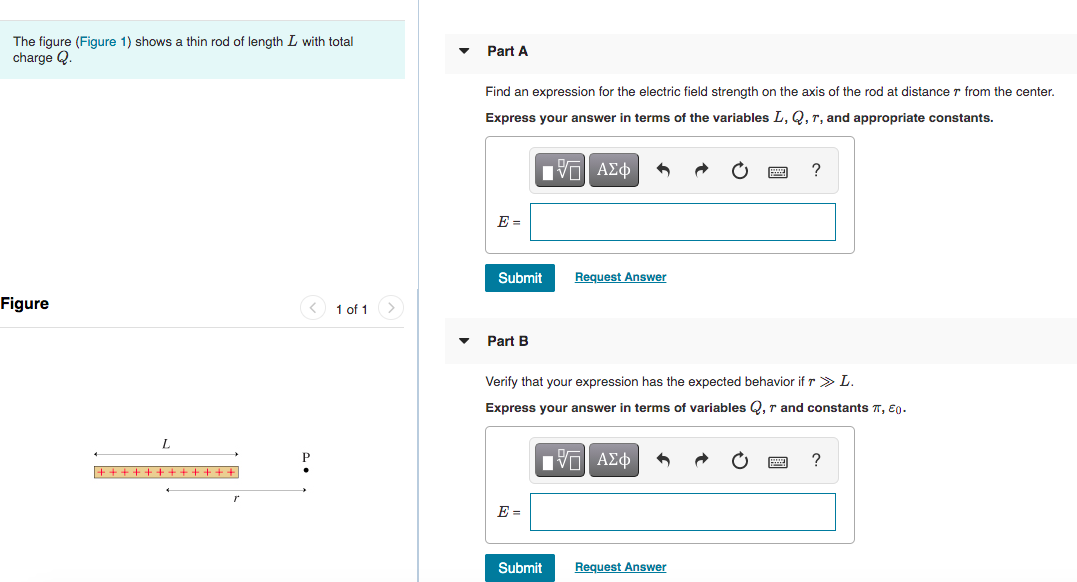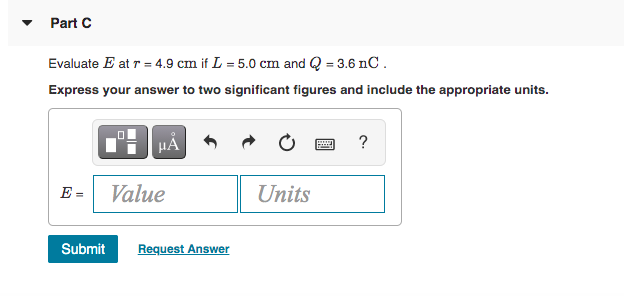Transcribed Image Text: The figure (Figure 1) shows a thin rod of length L with total charge Q. Part A Find an expression for the electric field strength on the axis of the rod at distance r from the center. Express your answer in terms of the variables L, Q, T, and appropriate constants. EVO AXO ? E = Submit Request Answer Figure < 1 of 1 > Part B Verify that your expression has the expected behavior if r >>L. Express your answer in terms of variables Q,r and constants , E0. L IVO ALO ? + + + + + + + + + + + + E = Submit Request Answer - Part C Evaluate E at r = 4.9 cm if L = 5.0 cm and Q = 3.6 nC. Express your answer to two significant figures and include the appropriate units. HÅ ? E = Value Units Submit Request Answer
More
Transcribed Image Text: The figure (Figure 1) shows a thin rod of length L with total charge Q. Part A Find an expression for the electric field strength on the axis of the rod at distance r from the center. Express your answer in terms of the variables L, Q, T, and appropriate constants. EVO AXO ? E = Submit Request Answer Figure < 1 of 1 > Part B Verify that your expression has the expected behavior if r >>L. Express your answer in terms of variables Q,r and constants , E0. L IVO ALO ? + + + + + + + + + + + + E = Submit Request Answer - Part C Evaluate E at r = 4.9 cm if L = 5.0 cm and Q = 3.6 nC. Express your answer to two significant figures and include the appropriate units. HÅ ? E = Value Units Submit Request Answer